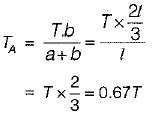Courses

# Test: Torsion of Shafts - 1

## 10 Questions MCQ Test GATE Civil Engineering (CE) 2022 Mock Test Series | Test: Torsion of Shafts - 1

Description
This mock test of Test: Torsion of Shafts - 1 for Civil Engineering (CE) helps you for every Civil Engineering (CE) entrance exam. This contains 10 Multiple Choice Questions for Civil Engineering (CE) Test: Torsion of Shafts - 1 (mcq) to study with solutions a complete question bank. The solved questions answers in this Test: Torsion of Shafts - 1 quiz give you a good mix of easy questions and tough questions. Civil Engineering (CE) students definitely take this Test: Torsion of Shafts - 1 exercise for a better result in the exam. You can find other Test: Torsion of Shafts - 1 extra questions, long questions & short questions for Civil Engineering (CE) on EduRev as well by searching above.
QUESTION: 1

### A solid shaft of circular cross-section is subjected to a torque T which produces a maximum shear stress fs in the shaft. The diameter of the shaft should be

Solution: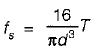∴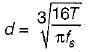QUESTION: 2

### A hollow shaft of inner radius 30 mm and outer radius 50 mm is subjected to a twisting moment. If the shear stress developed at inner radius of shaft is 60 N/mm2. What is the maximum shear stress in shaft?

Solution:

As we know, torsional shear stress varies linearly
∴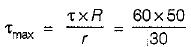= 100N/mm2

QUESTION: 3

### A solid steel shaft is surrounded by a copper shaft, such that,what is the ratio of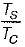if composite shaft is subjected to a twisting moment?

Solution: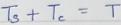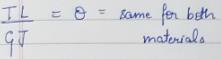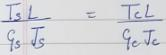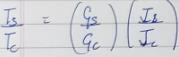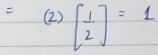QUESTION: 4

A shaft is subjected to a bending moment M and a torque T. The equivalent bending moment. Meq on the shaft is given by

Solution: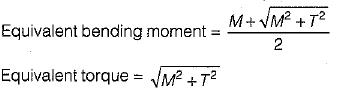QUESTION: 5

A shaft of diameter ‘d’ is subjected to bending moment M and twisting moment T. The developed principal stress will be

Solution: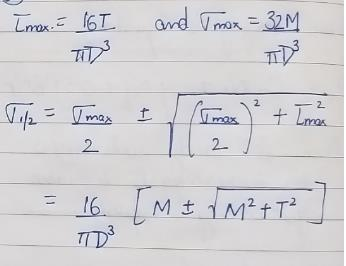QUESTION: 6

If n is the ratio of internal and external diameters of a hollow shaft, ratio of weights of hollow shaft and solid shaft in order to have same strength will be

Solution:

In order to have same strength,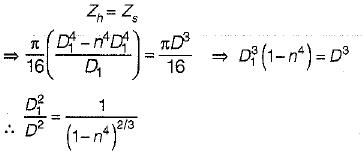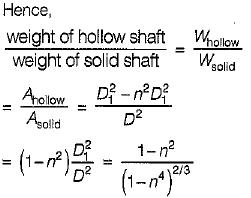QUESTION: 7

If a shaft is simultaneously subjected to a torque T and a bending moment M, the ratio of maximum bending stress and maximum shearing stress is given by

Solution:
QUESTION: 8

If two shafts of the same length, one of which is hollow, transmit equal torque and have equal maximum stress, then they should have equal

Solution: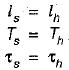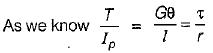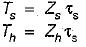As  Ts = Th,
∴ Zs = Zh

QUESTION: 9

A circular shaft fixed at A has diameter D for half of its length and diameter D/2 over the other half as shown in the figure. What is the rotation of C relative to B if the rotation of B relative to A is 0.1 radian?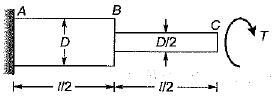Solution: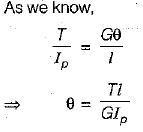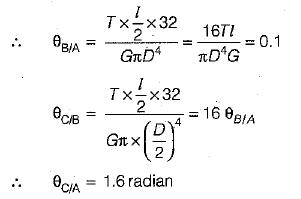QUESTION: 10

A shaft AS of'length l, diameter d is subjected to torque Tat section Csuch that AC = l/3, ends of the shaft are fixed, What is the resisting torque at A?

Solution:

Resisting torque at A, TA is given by,